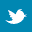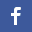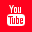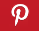Completely Solved C, C++ Programs Assignment.Quick Search Database Videos Tutorials Ebooks Forums FAQ Aboutus Household Industrial Manufacturing Service Shopping Transportation### C Program to print the second highest no. from a 1D matrix.

 Filed Under: C Assignments

PROBLEM STATEMENT:
Write a program to print the second highest no. from a 1D matrix.

THEORY:
The penultimate (one before last) number in an array, if it is arranged in an ascending order is the second highest number. If the highest number occurs more than once, then the location of the second highest number is (Size of array -1 - Number of times the highest number occurs) in the sorted array.

ALGORITHM:
Begin Set flag variable f to 0
Read n //Size of array Allocate size into array a for i ß0 to less than n, increment i Read a[i] //
Array inputs
//Initializing max1 with the greater of the two no.s, a[o] or a
If a is greater than a
max1ßa
max2ßa
else if ais greater than a
max1 ß a
max2 ß a
else
max2 ß a[n-1]
max1 ß a
//Loop to find out 2nd highest no.
For iß2 less than n, increment I by 1
if a[i] is equal to max1
continue with next iteration
if a[i] is greater than max1
fß1 //flag
max2 ß max1
max1 ß a[i]
else if a[i] is greater than max2
max2 ß a[i] f ß 1 //flag
//Printing
if f is equal to 0
Print ‘All the no.s are the same’
if f is equal to 1
Print ‘The second highest element is’ max2
End

PROGRAM LISTING:
//Program to print 2nd highest no. from a 1D array.
#include<stdio.h>
#include<conio.h>
#include<malloc.h>
void main()
{
int *a;
int n,i=0,max1,max2;
int f=0;//flag variable
clrscr(); //Accepting size of array from user
printf("\n Enter size of array :");
scanf("%d",&n);
a=(int*)malloc(n*sizeof(int)); //Accepting array elements from user
printf("Enter array elements \n");
for(i=0;i<n;i++)
scanf("%d",&a[i]); //Initializing max1 with the greater of the two no.s a[o] or a
if(a>a)
{
max1=a;
max2=a;
}//end of if else
if(a>a)
{
max1=a;
max2=a;
}//end of else
else
{
max2=a[n-1];
max1=a;
}//end of else
//Loop to find out 2nd highest no.
for(i=2;i<n;i++)
{ if(a[i]==max1)
continue;
if(a[i]>max1)
{
f=1;
max2=max1;
max1=a[i];
}//end of if else
if(a[i]>max2)
{
max2=a[i];
f=1;
}
}//end of for //Printing
if(f==0)
printf("All the no.s are the same");
if(f==1)
printf("The second highest element %d \n",max2);
}//end of main

OUTPUT:
Enter size of array :10
Enter array elements
11
The second highest element 8

DISCUSSION:
The max1 and max2 variables are not initialized with 0 or any other arbitrary number because a user might enter all the inputs as negative numbers, in that case the output shall be wrong. A flag variable need to be set in order to find out if all the numbers entered are the same, in that case there won’t be any second highest number.Back to main directory:Software Practical